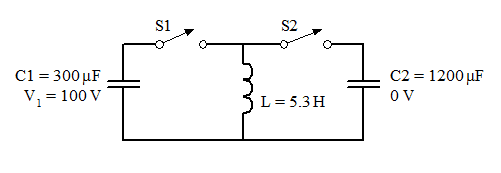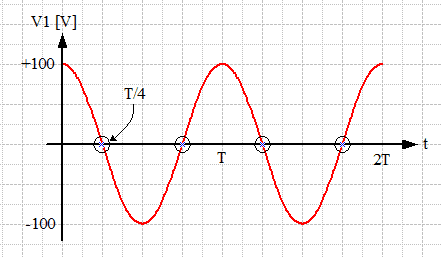# LC circuit and maximum voltage

• Archived
• KhaNgo
In summary, the 300 μF capacitor is initially charged to 100 V, the 1200 μF capacitor is uncharged, and the switches are both open. So the picture is two capapcitor in either side of the circuit. There is a inductor 5.3 H in the middle wire that divide the switches in eaither side of the circuit.a) What is the maximum voltage to which you can charge the 1200 microF by proper closing and opening of the two switches?b)How would you do it? Describe the sequence in which you would close and open switches and the times at which you would do so. The first switch is closed at .f

## Homework Statement

The 300 μF capacitor is initially charged to 100 V, the 1200 μF capacitor is uncharged, and the switches are both open. So the picture is two capapcitor in either side of the circuit. There is a inductor 5.3 H in the middle wire that divide the switches in eaither side of the circuit

a) What is the maximum voltage to which you can charge the 1200 microF by proper closing and opening of the two switches?
b)How would you do it? Describe the sequence in which you would close and open switches and the times at which you would do so. The first switch is closed at .

## Homework Equations

Q=Qnaught*coswt
I=Inaught*sinwt
w=sqaureroot of 1/LC

## The Attempt at a Solution

I tried to find the charge on the first one by using Q=VC and then try to find the total C of the circiut Ceq= C1+ C2. Then use the charge of 1 divide by the total Ceq
However, I can't figure out the right answer...
Any help will be greatly appreciated...
Thanks

Last edited:
A complete solution is offered:

Circuit diagram:Part (a): Maximum voltage we can put on C2
We can take advantage of the properties of an LC circuit to move all the energy stored in C1 to C2 (see part b). The energy stored on a capacitor is given by:

##E = \frac{1}{2} C V^2##

So then by conservation of energy we should find:

##\frac{1}{2} C2 V_2^2 = \frac{1}{2} C1 V_1^2##

Thus:

##V_2 = \sqrt{\frac{C1}{C2}} V_1 = \sqrt{\frac{300}{1200}} 100V = \frac{1}{2} 100V = 50 V##

Part (b): Switching strategy to place the maximum voltage on C2

The strategy will be to:
1. Close S1 forming an LC circuit comprising C1 and L. Sinusoidal oscillations will ensue with the energy available swapping back and forth between C1 and L.

2. Close S2 and open S1 at an instant where the voltage on C1 is zero. The energy stored in C1 will be zero at that time, having been transferred to the inductor and stored in its magnetic field. The new current path for the inductor current is now via C2, causing a new LC circuit to form containing all the energy that was initially stored in C1.

3. Open S2 at an instant when the current falls to zero in this new LC circuit, leaving C2 with all the energy and hence the maximum charge possible.

Note that this is considerably more energy than would be obtained by simply connecting the capacitors in parallel. That method would leave charge and energy distributed across both capacitors, and the same voltage on both. With the switching scenario described the first capacitor is left with zero charge and voltage, the inductor with zero current, and all the energy stored in the second capacitor.

The timings of the switchings can be determined from the natural frequencies of the two LC circuits, ##ω_o = \frac{1}{\sqrt{L C}}##. We have ##ω_1 = 25.08~rad/sec~~## and ##ω_2 = 12.34~rad/sec## respectively, the periods of the oscillations are thus ##T_1 = 250.54~ms~~## and ##T_2 = 501.08~ms~~## respectively.

In the first LC circuit capacitor C1 starts with its maximum voltage, so we can sketch the resulting voltage waveform as a cosine curve:Zero crossing occur at T/4, 3T/4, 5T/4,... and so on. So the first S1 switch opening / switch S2 closing opportunity occurs at time t = 62.64 ms after S1 is first closed.

The current in the second LC circuit begins on a maximum (all the energy stored in the inductor). So it also can be represented by a cosine curve. Again waiting for a zero crossing, for current this time, places the first S2 opening opportunity at T2/4 = 125.27 ms after S2 closed.

Note that you can choose the eventual polarity of the voltage across C2 by choosing an appropriate zero crossing. The current direction changes on every half cycle of the oscillation.

#### Attachments

•Greg Bernhardt
One problem that the above solution has is that it relies on being able to operate two switches simultaneously: Opening S1 and closing S2 at the same time. In practice this is difficult to achieve, and a minuscule timing error that briefly has both switches open while the inductor is carrying maximum current could lead to excessive potentials across the switch contacts and arcing. This would result in energy being lost.

An alternative switching sequence that avoids having to operate two switches simultaneously, and thus avoids the possibility of arcing at the switch contacts if the timing is not exact would be as follows:

1) Close S1. LC oscillations will ensue.
2) At an instant when the voltage on C1 is zero, close S2. Since all the energy will be safely stored in the inductor during this operation, none will be lost. This forms an LC circuit where the capacitors are in parallel.
3) Open switch S1 when the voltage on the combined capacitors is at a zero crossing. Capacitor C2 will still be in the circuit to absorb the inductor current while C1 is removed.
4) Open switch S2 when the inductor current makes a zero crossing. This again leaves all the energy on C2.

Timing calculations follow the same method as in the previous solution, the only difference being the natural frequency of the combined capacitor LC circuit will be lower, so the time to open S1 will be longer accordingly.

Last edited: